# Funding Areas

FOR MORE RESEARCH TOPICS

## Locality Problems of Massive Quantum Particles

The uncertainty principle implies that quantum theories cannot define a precise value for the location of a quantum particle. Hence, quantum functions yield an expression for the probability density of the particle’s location.

Fig. 1 depicts the decay modes of three motionless particles. For example, an electron-positron is one of the decay modes of theboson. The outgoing particles are detected by the pertinent devices and their position and momentum show that they emerged from a svery small space-time region. Therefore, in order to explain such a process, a quantum theory of a specific kind of particles should provide a consistent expression for spatial density.

Density is a mathematically real 0-component of a 4-vector (see  pp. 73-78; , pp. 23, 24) that has the dimension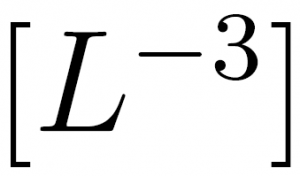. These properties impose restrictions on an acceptable expression for density. These constraints are relevant to the following discussion which examines quantum theories.

1. It is now recognized that the neutrino is a massive particle (see , p. 1307, ). Therefore, it is assumed here, that the neutrino is a Dirac particle (see item 2 below). The Dirac theory provides a consistent expression for density and for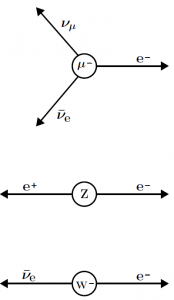Figure 1: Leptonic decay of particles (see text).

its associated 4-current(1)

(see , p. 24). Here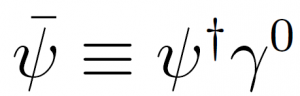The upper part of fig. 1 comprises only Dirac particles. Therefore, the Dirac theory explains density issues of muon decay.

1. Let us examine the Majorana theory of the neutrino. This theory uses four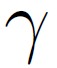matrices that comprise only pure imaginary numbers . Therefore, the function that solves the Majorana equation is real. However, the pure imaginary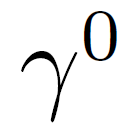means that the Majorana 4-current (1) is also pure imaginary. The Majorana theory is therefore inherently wrong, because it has no acceptable expression for density.

Experimental data support this result. For example, a neutrinoless double beta decay is a typical Majorana process. And indeed, in spite of many experimental efforts, “modern searches have found no evidence for the decay” .

1. Thedecay of fig. 1 shows two outgoing Dirac particles whose location has a well-defined theoretical expression. However, the electroweak literature does not show a consistent expression for theboson density.
2. Thedecay of fig. 1 shows two outgoing Dirac particles whose location has a well-defined theoretical expression. However, the electroweak literature does not show a consistent expression for theboson density.

Research topic #1: Can the density-related discrepancy of the Majorana theory be resolved?

Research topic #2: Can the density-related discrepancy of the electroweaktheory be resolved?

Research topic #3: Can the density-related discrepancy of the electroweaktheory be resolved?

Remark: A consistent expression for density is a crucial element of quantum mechanics as well as of quantum field theories. Indeed, a respectable textbook sustain with this claim. “First, some good news: quantum field theory is based on the same quantum mechanics that was invented by Schroedinger, Heisenberg, Pauli, Born, and others, in 1925-26, and has been used ever since in atomic, molecular, nuclear and condensed matter physics” (see , p. 49).

#### References

 L. D. Landau and E. M. Lifshitz The Classical Theory of Fields (Elsevier, Amsterdam, 2005).

 J. D. Bjorken and S.D. Drell, Relativistic Quantum Mechanics (McGraw-Hill, New York, 1964).

 J. A. Formaggio and G. P. Zeller, Rev. Mod. Phys. 84, 1307 (2012).

 http://pdg.lbl.gov/2018/reviews/rpp2018-rev-intro-neutrino-prop.pdf

 P. B. Pal, Am. J. Phys., 79, 485 (2011).

 https://en.wikipedia.org/wiki/Double_beta_decay#Status

 S.Weinberg, The Quantum Theory of Fields, Vol. I (Cambridge University Press, Cambridge, 1995).